### Home > MC1 > Chapter 9 > Lesson 9.1.1 > Problem9-7

9-7.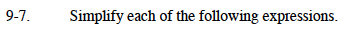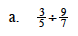Try using a Super Giant One to help you solve this problem, as shown in the box below.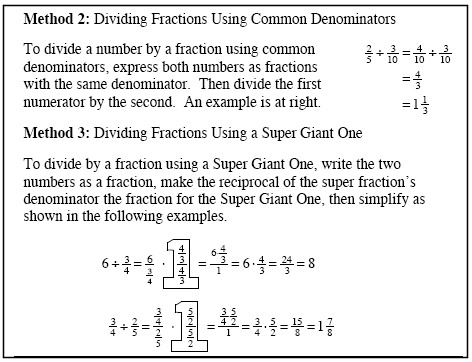Rewrite the problem, using a Super Giant One.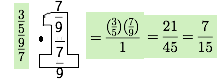Multiply by the Super Giant One to find the solution.

$\frac{7}{15}$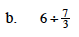$\text{Think of 6 as being the fraction }\frac{6}{1}.\text{ Complete the problem as shown in part (a).}$

$\frac{18}{7}=2\frac{4}{7}$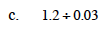$\text{You can express these numbers as fractions, rewriting this problem as } \frac{12}{10}\div\frac{3}{100}.$

Now try using the Super Giant One method as you did in part (a).

Another way to divide these numbers would be to think about how many groups of three hundredths would fit into one and two-tenths.
Remember that one and two-tenths is the same as 120-hundredths.
So how many groups of three-hundredths fit into 120-hundredths?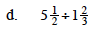You may want to change these mixed numbers to fractions greater than one.
After doing so, the steps are the same as those in part (a).